SLASEG8A March   2016  – July 2017

PRODUCTION DATA.

1. Features
2. Applications
3. Description
4. Revision History
5. Device Comparison Table
6. Pin Configuration and Functions
7. Specifications
8. Parametric Measurement Information
9. Detailed Description
1. 9.1 Overview
2. 9.2 Functional Block Diagram
3. 9.3 Feature Description
1. 9.3.1 Power-on-Reset (POR) Function
2. 9.3.2 Device Clocking
3. 9.3.3 Serial Audio Port
4. 9.3.4 Enable Device
5. 9.3.5 Volume Control
1. 9.3.5.1 DAC Digital Gain Control
6. 9.3.6 Adjustable Amplifier Gain and Switching Frequency Selection
7. 9.3.7 Error Handling and Protection Suite
8. 9.3.8 GPIO Port and Hardware Control Pins
9. 9.3.9 I2C Communication Port
4. 9.4 Device Functional Modes
10. 10Application and Implementation
1. 10.1 Application Information
2. 10.2 Typical Applications
1. 10.2.1 2.0 (Stereo BTL) System
2. 10.2.2 Mono (PBTL) Systems
3. 10.2.3 2.1 (Stereo BTL + External Mono Amplifier) Systems
11. 11Power Supply Recommendations
1. 11.1 Power Supplies
12. 12Layout
1. 12.1 Layout Guidelines
1. 12.1.1 General Guidelines for Audio Amplifiers
2. 12.1.2 Importance of PVDD Bypass Capacitor Placement on PVDD Network
3. 12.1.3 Optimizing Thermal Performance
1. 12.1.3.1 Device, Copper, and Component Layout
2. 12.1.3.2 Stencil Pattern
1. 12.1.3.2.1 PCB footprint and Via Arrangement
2. 12.2 Layout Example
13. 13Register Maps
1. 13.1 Registers - Page 0
2. 13.2 Registers - Page 1
14. 14Device and Documentation Support
15. 15Mechanical, Packaging, and Orderable Information

• DCA|48
• DCA|48

7 Specifications

7.1 Absolute Maximum Ratings

Free-air room temperature 25°C (unless otherwise noted)(1)
MIN MAX UNIT
DVDD, AVDD, CPVDD Low-voltage digital, analog, charge pump supply –0.3 3.9 V
PVDD PVDD supply –0.3 30 V
VI(AmpCtrl) Input voltage for SPK_GAIN/FREQ and SPK_FAULT pins –0.3 VGVDD + 0.3 V
VI(DigIn) DVDD referenced digital inputs(2) –0.5 VDVDD + 0.5 V
VI(SPK_OUTxx) Voltage at speaker output pins –0.3 32 V
Ambient operating temperature, TA –25 85 °C
TJ Operating junction temperature, digital die –40 125 °C
Operating junction temperature, power die –40 165 °C
Tstg Storage temperature –40 125 °C
Stresses beyond those listed under Absolute Maximum Ratings may cause permanent damage to the device. These are stress ratings only, and functional operation of the device at these or any other conditions beyond those indicated under Recommended Operating Conditions is not implied. Exposure to absolute-maximum-rated conditions for extended periods may affect device reliability.
DVDD referenced digital pins include: ADR0, ADR1, GPIO0, GPIO2, LRCK/FS, MCLK, RESET, SCL, SCLK, SDA, SDIN, and SPK_MUTE.

7.2 ESD Ratings

VALUE UNIT
V(ESD) Electrostatic discharge Human-body model (HBM), per ANSI/ESDA/JEDEC JS-001(1) ±2000 V
Charged-device model (CDM), per JEDEC specification JESD22-C101(2) ±500
JEDEC document JEP155 states that 2000-V HBM allows safe manufacturing with a standard ESD control process.
JEDEC document JEP157 states that 500-V CDM allows safe manufacturing with a standard ESD control process.

7.3 Recommended Operating Conditions

Free-air room temperature 25°C (unless otherwise noted)
MIN NOM MAX UNIT
V(POWER) Power supply inputs DVDD, AVDD, CPVDD 2.9 3.63 V
PVDD 4.5 26.4
RSPK Minimum speaker load BTL Mode 3 Ω
PBTL Mode 2 Ω
VIH(DigIn) Input logic high for DVDD referenced digital inputs(2)(1) 0.9 × VDVDD VDVDD V
VIL(DigIn) Input logic low for DVDD referenced digital inputs(2)(2) VDVDD 0 0.1 × VDVDD V
LOUT Minimum inductor value in LC filter under short-circuit condition 1 4.7 µH
The best practice for driving the input pins of the TAS5782M device is to power the drive circuit or pullup resistor from the same supply which provides the DVDD power supply.
The best practice for driving the input pins of the TAS5782M device low is to pull them down, either actively or through pulldown resistors to the system ground.

7.4 Thermal Information

THERMAL METRIC(1) TAS5782M
DCA (TSSOP)
48 PINS
UNIT
JEDEC
STANDARD
2-LAYER PCB
JEDEC
STANDARD
4-LAYER PCB
TAS5782MEVM
4-LAYER PCB
RθJA Junction-to-ambient thermal resistance 41.8 27.6 19.4 °C/W
RθJC(top) Junction-to-case (top) thermal resistance 14.4 14.4 14.4 °C/W
RθJB Junction-to-board thermal resistance 9.4 9.4 9.4 °C/W
ψJT Junction-to-top characterization parameter 0.6 0.6 2 °C/W
ψJB Junction-to-board characterization parameter 8.1 9.3 4.8 °C/W
RθJC(bot) Junction-to-case (bottom) thermal resistance N/A N/A N/A °C/W

7.5 Electrical Characteristics

Free-air room temperature 25°C (unless otherwise noted) Measurements were made using TAS5782MEVM board and Audio Precision System 2722 with Analog Analyzer filter set to 40 kHz brickwall filter. The device output PWM frequency was set to 768 kHz unless otherwise noted.
PARAMETER TEST CONDITIONS MIN TYP MAX UNIT
DIGITAL I/O
|IIH|1 Input logic high current level for DVDD referenced digital input pins(2) VIN(DigIn) = VDVDD 10 µA
|IIL|1 Input logic low current level for DVDD referenced digital input pins(2) VIN(DigIn) = 0 V –10 µA
VIH1 Input logic high threshold for DVDD referenced digital inputs(2) 70% VDVDD
VIL1 Input logic low threshold for DVDD referenced digital inputs(2) 30% VDVDD
VOH(DigOut) Output logic high voltage level(2) IOH = 4 mA 80% VDVDD
VOL(DigOut) Output logic low voltage level(2) IOH = –4 mA 22% VDVDD
VOL(SPK_FAULT) Output logic low voltage level for SPK_FAULT With 100-kΩ pullup resistor 0.8 V
GVDD_REG GVDD regulator voltage 7 V
I2C CONTROL PORT
CL(I2C) Allowable load capacitance for each I2C Line 400 pF
fSCL(fast) Support SCL frequency No wait states, fast mode 400 kHz
fSCL(slow) Support SCL frequency No wait states, slow mode 100 kHz
VNH Noise margin at High level for each connected device (including hysteresis) 0.2 × VDD V
MCLK AND PLL SPECIFICATIONS
DMCLK Allowable MCLK duty cycle 40% 60%
fMCLK Supported MCLK frequencies Up to 50 MHz 128 512 fS(1)
fPLL PLL input frequency Clock divider uses fractional divide
D > 0, P = 1
6.7 20 MHz
Clock divider uses integer divide
D = 0, P = 1
1 20
SERIAL AUDIO PORT
tDLY Required LRCK/FS to SCLK rising edge delay 5 ns
DSCLK Allowable SCLK duty cycle 40% 60%
fS Supported input sample rates 8 96 kHz
fSCLK Supported SCLK frequencies 32 64 fS(1)
fSCLK SCLK frequency Either master mode or slave mode 24.576 MHz
SPEAKER AMPLIFIER (ALL OUTPUT CONFIGURATIONS)
AV(SPK_AMP) Speaker amplifier gain SPK_GAIN/FREQ voltage < 3 V,
see Adjustable Amplifier Gain and Switching Frequency Selection
20 dBV
SPK_GAIN/FREQ voltage > 3.3 V,
see Adjustable Amplifier Gain and Switching Frequency Selection
26
ΔAV(SPK_AMP) Typical variation of speaker amplifier gain ±1 dBV
fSPK_AMP Switching frequency of the speaker amplifier Switching frequency depends on voltage presented at SPK_GAIN/FREQ pin and the clocking arrangement, including the incoming sample rate, see Adjustable Amplifier Gain and Switching Frequency Selection 176.4 768 kHz
KSVR Power supply rejection ratio Injected Noise = 50 Hz to 60 Hz, 200 mVP-P, Gain = 26 dB, input audio signal = digital zero 60 dB
rDS(on) Drain-to-source on resistance of the individual output MOSFETs VPVDD = 24 V, I(SPK_OUT) = 500 mA, TJ = 25°C, includes PVDD/PGND pins, leadframe, bondwires and metallization layers. 120
VPVDD = 24 V, I(SPK_OUT) = 500 mA, TJ = 25°C 90
OCETHRES SPK_OUTxx overcurrent error threshold 7.5 A
OTETHRES Overtemperature error threshold 165 °C
OCECLRTIME Time required to clear overcurrent error after error condition is removed. 1.3 s
OTECLRTIME Time required to clear overtemperature error after error condition is removed. 1.3 s
OVETHRES(PVDD) PVDD overvoltage error threshold 27 V
UVETHRES(PVDD) PVDD undervoltage error threshold 4.3 V
SPEAKER AMPLIFIER (STEREO BTL)
|VOS| Amplifier offset voltage Measured differentially with zero input data, SPK_GAIN/FREQ pin configured for 20 dB gain, VPVDD = 12 V 2 mV
Measured differentially with zero input data, SPK_GAIN/FREQ pin configured for 26 dB gain, VPVDD = 24 V 5 15
ICN(SPK) Idle channel noise VPVDD = 12 V, SPK_GAIN = 20 dB, RSPK = 8 Ω, A-Weighted 49 µVRMS
VPVDD = 15 V, SPK_GAIN = 20 dB, RSPK = 8 Ω, A-Weighted 59
VPVDD = 19 V, SPK_GAIN = 26 dB, RSPK = 8 Ω, A-Weighted 81
VPVDD = 24 V, SPK_GAIN = 26 dB, RSPK = 8 Ω, A-Weighted 82
PO(SPK) Output Power (Per Channel) VPVDD = 12 V, SPK_GAIN = 20 dB, RSPK = 4 Ω, THD+N = 0.1% 14 W
VPVDD = 12 V, SPK_GAIN = 20 dB, RSPK = 8 Ω, THD+N = 0.1% 8
VPVDD = 15 V, SPK_GAIN = 26 dB, RSPK = 4 Ω, THD+N = 0.1% 23
VPVDD = 15 V, SPK_GAIN = 26 dB, RSPK = 8 Ω, THD+N = 0.1% 13
VPVDD = 19 V, SPK_GAIN = 26 dB, RSPK = 4 Ω, THD+N = 0.1% 34
VPVDD = 19 V, SPK_GAIN = 26 dB, RSPK = 8 Ω, THD+N = 0.1% 20
VPVDD = 24 V, SPK_GAIN = 26 dB, RSPK = 4 Ω, THD+N = 0.1% 40
VPVDD = 24 V, SPK_GAIN = 26 dB, RSPK = 8 Ω, THD+N = 0.1% 33
SNR Signal-to-noise ratio (referenced to 0 dBFS input signal) VPVDD = 12 V, SPK_GAIN = 20 dB, RSPK = 8 Ω, A-Weighted, –120 dBFS Input 103 dB
VPVDD = 15 V, SPK_GAIN = 26 dB, RSPK = 8 Ω, A-Weighted, –120 dBFS Input 102
VPVDD = 19 V, SPK_GAIN = 26 dB, RSPK = 8 Ω, A-Weighted, –120 dBFS Input 103
VPVDD = 24 V, SPK_GAIN = 26 dB, RSPK = 8 Ω, A-Weighted, –120 dBFS Input 105
THD+NSPK Total harmonic distortion and noise VPVDD = 12 V, SPK_GAIN = 20 dB, RSPK = 4 Ω, PO = 1 W, f = 1kHz 0.021%
VPVDD = 12 V, SPK_GAIN = 20 dB, RSPK = 8 Ω, PO = 1 W, f = 1kHz 0.022%
VPVDD = 15 V, SPK_GAIN = 26 dB, RSPK = 4 Ω, PO = 1 W, f = 1kHz 0.02%
VPVDD = 15 V, SPK_GAIN = 26 dB, RSPK = 8 Ω, PO = 1 W, f = 1kHz 0.037%
VPVDD = 19 V, SPK_GAIN = 26 dB, RSPK = 4 Ω, PO = 1 W, f = 1kHz 0021%
VPVDD = 19 V, SPK_GAIN = 26 dB, RSPK = 8 Ω, PO = 1 W, f = 1kHz 0.028%
VPVDD = 24 V, SPK_GAIN = 26 dB, RSPK = 4 Ω, PO = 1 W, f = 1kHz 0.027%
VPVDD = 24 V, SPK_GAIN = 26 dB, RSPK = 8 Ω, PO = 1 W, f = 1kHz 0.038%
X-talkSPK Cross-talk (worst case between left-to-right and right-to-left coupling) VPVDD = 12 V, SPK_GAIN = 20 dB, RSPK = 8 Ω, Input Signal 250 mVrms,
1-kHz Sine, across f(S)
–90 dB
VPVDD = 15 V, SPK_GAIN = 26 dBV, RSPK = 8 Ω, Input Signal 250 mVrms,
1-kHz Sine, across f(S)
–102
VPVDD = 19 V, SPK_GAIN = 26 dBV, RSPK = 8 Ω, Input Signal 250 mVrms,
1-kHz Sine, across f(S)
–93
VPVDD = 24 V, SPK_GAIN = 26 dBV, RSPK = 8 Ω, Input Signal 250 mVrms,
1-kHz Sine, across f(S)
–93
SPEAKER AMPLIFIER (MONO PBTL)
|VOS| Amplifier offset voltage Measured differentially with zero input data, SPK_GAIN/FREQ pin configured for 20 dB gain, VPVDD = 12 V 0.7 mV
Measured differentially with zero input data, SPK_GAIN/FREQ pin configured for 26 dB gain, VPVDD = 24 V 4
ICN Idle channel noise VPVDD = 12 V, SPK_GAIN = 20 dB, RSPK = 8 Ω, A-Weighted 48 µVRMS
VPVDD = 15 V, SPK_GAIN = 20 dB, RSPK = 8 Ω, A-Weighted 49
VPVDD = 19 V, SPK_GAIN = 26 dB, RSPK = 8 Ω, A-Weighted 83
VPVDD = 24 V, SPK_GAIN = 26 dB, RSPK = 8 Ω, A-Weighted 82
PO Output power (per channel) VPVDD = 12 V, SPK_GAIN = 20 dB, RSPK = 2 Ω, THD+N = 0.1%, Unless otherwise noted 30 W
VPVDD = 12 V, SPK_GAIN = 20 dB, RSPK = 4 Ω, THD+N = 0.1%, Unless otherwise noted 16
VPVDD = 12 V, SPK_GAIN = 20 dB, RSPK = 8 Ω, THD+N = 0.1% 9
VPVDD = 15 V, SPK_GAIN = 26 dB, RSPK = 2 Ω, THD+N = 0.1%, Unless otherwise noted 44
VPVDD = 15 V, SPK_GAIN = 26 dB, RSPK = 4 Ω, THD+N = 0.1%, Unless otherwise noted 22
VPVDD = 15 V, SPK_GAIN = 26 dB, RSPK = 8 Ω, THD+N = 0.1% 13
VPVDD = 19 V, SPK_GAIN = 26 dB, RSPK = 2 Ω, THD+N = 0.1%, Unless otherwise noted 50
VPVDD = 19 V, SPK_GAIN = 26 dB, RSPK = 4 Ω, THD+N = 0.1%, Unless otherwise noted 36
VPVDD = 19 V, SPK_GAIN = 26 dB, RSPK = 8 Ω, THD+N = 0.1% 20
VPVDD = 24 V, SPK_GAIN = 26 dB, RSPK = 2 Ω, THD+N = 0.1%, Unless otherwise noted 40
VPVDD = 24 V, SPK_GAIN = 26 dB, RSPK = 4 Ω, THD+N = 0.1%, Unless otherwise noted 61
VPVDD = 24 V, SPK_GAIN = 26 dB, RSPK = 8 Ω, THD+N = 0.1% 34
SNR Signal-to-noise ratio
(referenced to 0 dBFS input signal)
VPVDD = 12 V, SPK_GAIN = 20 dB, RSPK = 8 Ω, A-Weighted, –120 dBFS Input 105 dB
VPVDD = 15 V, SPK_GAIN = 26 dB, RSPK = 8 Ω, A-Weighted, –120 dBFS Input 104
VPVDD = 19 V, SPK_GAIN = 26 dB, RSPK = 8 Ω, A-Weighted, –120 dBFS Input 105
VPVDD = 24 V, SPK_GAIN = 26 dB, RSPK = 8 Ω, A-Weighted, –120 dBFS Input 107
THD+N Total harmonic distortion and noise VPVDD = 12 V, SPK_GAIN = 20 dB, RSPK = 2 Ω, PO = 1 W, f = 1kHz 0.014%
VPVDD = 12 V, SPK_GAIN = 20 dB, RSPK = 4 Ω, PO = 1 W, f = 1kHz 0.011%
VPVDD = 12 V, SPK_GAIN = 20 dB, RSPK = 8 Ω, PO = 1 W, f = 1kHz 0.014%
VPVDD = 15 V, SPK_GAIN = 26 dB, RSPK = 2 Ω, PO = 1 W, f = 1kHz 0.015%
VPVDD = 15 V, SPK_GAIN = 26 dB, RSPK = 4 Ω, PO = 1 W, f = 1kHz 0.013%
VPVDD = 15 V, SPK_GAIN = 26 dB, RSPK = 8 Ω, PO = 1 W, f = 1kHz 0.015%
VPVDD = 19 V, SPK_GAIN = 26 dB, RSPK = 2 Ω, PO = 1 W, f = 1kHz 0.018%
V, RSPK = 4 Ω, PO = 1 W, f = 1kHz 0.012%
VPVDD = 19 V, SPK_GAIN = 26 dB, RSPK = 8 Ω, PO = 1 W, f = 1kHz 0.020%
VPVDD = 24 V, SPK_GAIN = 26 dB, RSPK = 2 Ω, PO = 1 W, f = 1kHz 0.028%
VPVDD = 24 V, SPK_GAIN = 26 dB, RSPK = 4 Ω, PO = 1 W, f = 1kHz 0.02%
VPVDD = 24 V, SPK_GAIN = 26 dB, RSPK = 8 Ω, PO = 1 W, f = 1kHz 0.027%
A unit of fS indicates that the specification is the value listed in the table multiplied by the sample rate of the audio used in the TAS5782M device.
DVDD referenced digital pins include: ADR0, ADR1, GPIO0, GPIO2, LRCK/FS, MCLK,RESET, SCL, SCLK, SDA, SDIN, and SPK_MUTE.

7.6 Power Dissipation Characteristics

Free-air room temperature 25°C (unless otherwise noted)
VPVDD
(V)
SPK_GAIN(1)(2)(3)
(dBV)
fSPK_AMP
(kHz)
STATE OF
OPERATION
RSPK
(Ω)
IPVDD(4)
(mA)
IDVDD(5)
(mA)
PDISS
(W)
7.4 20 384 Idle 4 21.30 59.70 0.355
6 21.33 59.68 0.355
8 21.30 59.70 0.355
Mute 4 21.33 58.82 0.352
6 21.34 58.81 0.352
8 21.36 58.81 0.352
Standby 4 2.08 12.41 0.056
6 2.11 12.41 0.057
8 2.17 12.41 0.057
Powerdown 4 2.03 0.730 0.017
6 2.04 0.740 0.018
8 2.06 0.740 0.018
768 Idle 4 27.48 59.7 0.400
6 27.49 59.73 0.401
8 24.46 59.72 0.378
Mute 4 27.50 58.8 0.398
6 27.51 58.8 0.398
8 27.52 58.81 0.398
Standby 4 2.04 12.41 0.056
6 2.08 12.41 0.056
8 2.11 12.41 0.057
Powerdown 4 2.06 0.73 0.018
6 2.07 0.74 0.018
8 2.08 0.74 0.018
11.1 20 384 Idle 4 24.33 59.74 0.467
6 24.32 59.74 0.467
8 24.36 59.70 0.467
Mute 4 24.36 58.81 0.464
6 24.32 58.82 0.464
8 24.37 58.84 0.465
Standby 4 3.58 12.40 0.081
6 3.57 12.41 0.081
8 3.58 12.42 0.081
Powerdown 4 3.52 0.74 0.042
6 3.52 0.74 0.042
8 3.54 0.74 0.042
768 Idle 4 30.70 59.70 0.538
6 30.65 59.72 0.537
8 30.67 59.71 0.537
Mute 4 3.072 58.80 0.528
6 30.69 58.81 0.535
8 30.69 58.81 0.535
Standby 4 3.54 12.40 0.080
6 3.54 12.41 0.080
8 3.58 12.42 0.081
Powerdown 4 3.53 0.74 0.042
6 3.53 0.74 0.042
8 3.55 0.74 0.042
12 20 384 Idle 4 25.07 59.72 0.498
6 25.08 59.73 0.498
8 25.10 59.71 0.498
Mute 4 25.12 58.84 0.496
6 25.08 58.82 0.495
8 25.11 58.82 0.495
Standby 4 3.92 12.40 0.088
6 3.93 12.41 0.088
8 3.94 12.41 0.088
Powerdown 4 3.87 0.75 0.049
6 3.85 0.74 0.049
8 3.87 0.75 0.049
768 Idle 4 31.31 59.72 0.573
6 31.29 59.71 0.573
8 31.31 59.74 0.573
Mute 4 31.31 58.80 0.570
6 31.33 58.81 0.570
8 31.32 58.81 0.570
Standby 4 3.88 12.40 0.087
6 3.90 12.41 0.088
8 3.91 12.41 0.088
Powerdown 4 3.89 0.75 0.049
6 3.91 0.74 0.049
8 3.88 0.75 0.049
15 26 384 Idle 4 27.94 59.73 0.616
6 27.91 59.75 0.616
8 27.75 59.69 0.613
Mute 4 27.98 58.84 0.614
6 27.94 58.87 0.613
8 27.88 58.85 0.612
Standby 4 5.09 12.41 0.117
6 5.12 12.41 0.118
8 5.19 12.41 0.119
Powerdown 4 5.02 0.74 0.078
6 5.06 0.74 0.078
8 5.14 0.74 0.080
768 Idle 4 33.05 59.7 0.693
6 33.03 59.72 0.693
8 33.08 59.68 0.693
Mute 4 33.03 58.81 0.690
6 33.04 58.81 0.690
8 33.05 58.80 0.690
Standby 4 5.07 12.41 0.117
6 5.09 12.41 0.117
8 5.14 12.41 0.118
Powerdown 4 5.02 0.74 0.078
6 5.04 0.74 0.078
8 5.09 0.74 0.079
19.6 26 384 Idle 4 32.27 59.77 0.830
6 32.19 59.76 0.828
8 32.08 59.75 0.826
Mute 4 32.27 58.85 0.827
6 32.24 58.87 0.826
8 32.22 58.86 0.826
Standby 4 6.95 12.40 0.177
6 6.93 12.42 0.177
8 7.00 12.41 0.178
Powerdown 4 6.89 0.74 0.137
6 6.90 0.74 0.138
8 6.96 0.73 0.139
768 Idle 4 34.99 59.74 0.883
6 34.95 59.74 0.882
8 34.97 59.71 0.882
Mute 4 34.96 58.85 0.879
6 34.98 58.83 0.880
8 34.96 58.81 0.879
Standby 4 6.93 12.40 0.177
6 6.93 12.42 0.177
8 6.98 12.41 0.178
Powerdown 4 6.84 0.74 0.137
6 6.89 0.74 0.137
8 6.90 0.73 0.138
24 26 384 Idle 4 36.93 59.80 1.084
6 36.87 59.81 1.082
8 36.77 59.76 1.080
Mute 4 36.94 58.91 1.081
6 36.89 58.89 1.080
8 36.85 58.90 1.079
Standby 4 8.73 12.40 0.250
6 8.72 12.40 0.250
8 8.71 12.40 0.250
Powerdown 4 8.64 0.74 0.210
6 8.66 0.74 0.210
8 8.69 0.73 0.211
768 Idle 4 36.84 59.73 1.081
6 36.86 59.76 1.082
8 36.83 59.78 1.081
Mute 4 36.85 58.85 1.079
6 36.84 58.84 1.078
8 36.82 58.83 1.078
Standby 4 8.66 12.40 0.249
6 8.68 12.40 0.249
8 8.71 12.40 0.250
Powerdown 4 8.63 0.74 0.210
6 8.64 0.74 0.210
8 8.65 0.73 0.210
Mute: B0-P0-R3-D0,D4 = 1
Standby: B0-P0-R2-D4 = 1
Power down: B0-P0-R2-D0 = 1
IPVDD refers to all current that flows through the PVDD supply for the DUT. Any other current sinks not directly related to the DUT current draw were removed.
IDVDD refers to all current that flows through the DVDD (3.3-V) supply for the DUT. Any other current sinks not directly related to the DUT current draw were removed.

7.7 MCLK Timing

See Figure 18.
MIN NOM MAX UNIT
tMCLK MCLK period 20 1000 ns
tMCLKH MCLK pulse width, high 9 ns
tMCLKL MCLK pulse width, low 9 ns

7.8 Serial Audio Port Timing – Slave Mode

See Figure 19.
MIN NOM MAX UNIT
fSCLK SCLK frequency 1.024 MHz
tSCLK SCLK period 40 ns
tSCLKL SCLK pulse width, low 16 ns
tSCLKH SCLK pulse width, high 16 ns
tSL SCLK rising to LRCK/FS edge 8 ns
tLS LRCK/FS Edge to SCLK rising edge 8 ns
tSU Data setup time, before SCLK rising edge 8 ns
tDH Data hold time, after SCLK rising edge 8 ns
tDFS Data delay time from SCLK falling edge 15 ns

7.9 Serial Audio Port Timing – Master Mode

See Figure 20.
MIN NOM MAX UNIT
tSCLK SCLK period 40 ns
tSCLKL SCLK pulse width, low 16 ns
tSCLKH SCLK pulse width, high 16 ns
tLRD LRCK/FS delay time from to SCLK falling edge –10 20 ns
tSU Data setup time, before SCLK rising edge 8 ns
tDH Data hold time, after SCLK rising edge 8 ns
tDFS Data delay time from SCLK falling edge 15 ns

7.10 I2C Bus Timing – Standard

MIN MAX UNIT
fSCL SCL clock frequency 100 kHz
tBUF Bus free time between a STOP and START condition 4.7 µs
tLOW Low period of the SCL clock 4.7 µs
tHI High period of the SCL clock 4 µs
tRS-SU Setup time for (repeated) START condition 4.7 µs
tS-HD Hold time for (repeated) START condition 4 µs
tD-SU Data setup time 250 ns
tD-HD Data hold time 0 900 ns
tSCL-R Rise time of SCL signal 20 + 0.1CB 1000 ns
tSCL-R1 Rise time of SCL signal after a repeated START condition and after an acknowledge bit 20 + 0.1CB 1000 ns
tSCL-F Fall time of SCL signal 20 + 0.1CB 1000 ns
tSDA-R Rise time of SDA signal 20 + 0.1CB 1000 ns
tSDA-F Fall time of SDA signal 20 + 0.1CB 1000 ns
tP-SU Setup time for STOP condition 4 µs

7.11 I2C Bus Timing – Fast

See Figure 21.
MIN MAX UNIT
fSCL SCL clock frequency 400 kHz
tBUF Bus free time between a STOP and START condition 1.3 µs
tLOW Low period of the SCL clock 1.3 µs
tHI High period of the SCL clock 600 ns
tRS-SU Setup time for (repeated)START condition 600 ns
tRS-HD Hold time for (repeated)START condition 600 ns
tD-SU Data setup time 100 ns
tD-HD Data hold time 0 900 ns
tSCL-R Rise time of SCL signal 20 + 0.1CB 300 ns
tSCL-R1 Rise time of SCL signal after a repeated START condition and after an acknowledge bit 20 + 0.1CB 300 ns
tSCL-F Fall time of SCL signal 20 + 0.1CB 300 ns
tSDA-R Rise time of SDA signal 20 + 0.1CB 300 ns
tSDA-F Fall time of SDA signal 20 + 0.1CB 300 ns
tP-SU Setup time for STOP condition 600 ns
tSP Pulse width of spike suppressed 50 ns

7.12 SPK_MUTE Timing

See Figure 22.
MIN MAX UNIT
tr Rise time 20 ns
tf Fall time 20 nsFigure 18. Timing Requirements for MCLK Input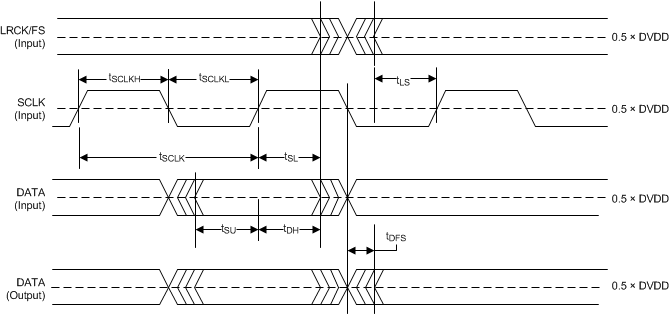Figure 19. Serial Audio Port Timing in Slave Mode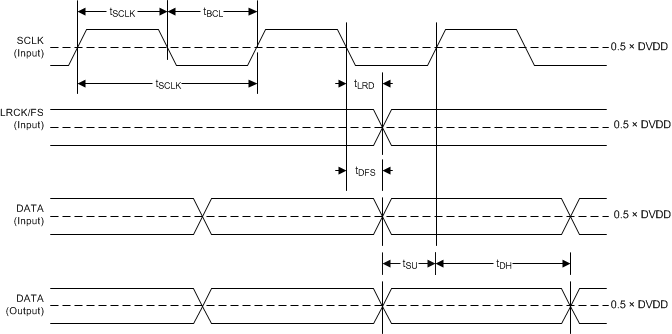Figure 20. Serial Audio Port Timing in Master Mode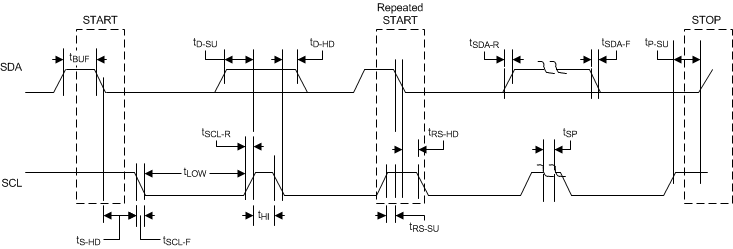Figure 21. I2C Communication Port Timing Diagram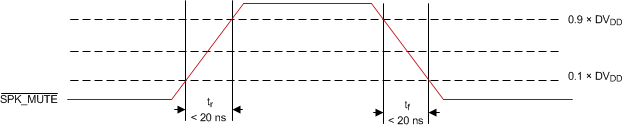Figure 22. SPK_MUTE Timing Diagram for Soft Mute Operation via Hardware Pin

7.13 Typical Characteristics

All performance plots were taken using the TAS5782MEVM Board at room temperature, unless otherwise noted. The term "traditional LC filter" refers to the output filter that is present by default on the TAS5782MEVM Board.

Table 1. Quick Reference Table

OUTPUT
CONFIGURATIONS
PLOT TITLE FIGURE NUMBER
Bridge Tied Load (BTL) Configuration Curves Frequency Response Figure 42
Output Power vs PVDD Figure 23
THD+N vs Frequency, VPVDD = 12 V Figure 24
THD+N vs Frequency, VPVDD = 15 V Figure 25
THD+N vs Frequency, VPVDD = 18 V Figure 26
THD+N vs Frequency, VPVDD = 24 V Figure 27
THD+N vs Power, VPVDD = 12 V Figure 28
THD+N vs Power, VPVDD = 15 V Figure 29
THD+N vs Power, VPVDD = 18 V Figure 30
THD+N vs Power, VPVDD = 24 V Figure 31
Idle Channel Noise vs PVDD Figure 32
Efficiency vs Output Power Figure 33
Efficiency vs Output Power Figure 34
Efficiency vs Output Power Figure 35
Idle Current Draw (Filterless) vs PVDD Figure 36
Crosstalk vs. Frequency Figure 37
PVDD PSRR vs Frequency Figure 38
DVDD PSRR vs Frequency Figure 39
AVDD PSRR vs Frequency Figure 40
CPVDD PSRR vs Frequency Figure 41
THD+N vs Frequency, VPVDD = 12 V Figure 43
THD+N vs Frequency, VPVDD = 15 V Figure 44
THD+N vs Frequency, VPVDD = 18 V Figure 45
THD+N vs Frequency, VPVDD = 24 V Figure 46
Parallel Bridge Tied Load (PBTL) Configuration Output Power vs PVDD Figure 47
THD+N vs Frequency, VPVDD = 12 V Figure 48
THD+N vs Frequency, VPVDD = 15 V Figure 49
THD+N vs Frequency, VPVDD = 18 V Figure 50
THD+N vs Frequency, VPVDD = 24 V Figure 51
THD+N vs Power, VPVDD = 12 V Figure 52
THD+N vs Power, VPVDD = 15 V Figure 53
THD+N vs Power, VPVDD = 18 V Figure 54
THD+N vs Power, VPVDD = 24 V Figure 55
Idle Channel Noise vs PVDD Figure 56
Efficiency vs Output Power Figure 57
THD+N vs Frequency, VPVDD = 12 V Figure 60
THD+N vs Frequency, VPVDD = 15 V Figure 61
THD+N vs Frequency, VPVDD = 18 V Figure 62
THD+N vs Frequency, VPVDD = 24 V Figure 63

7.13.1 Bridge Tied Load (BTL) Configuration Curves

Free-air room temperature 25°C (unless otherwise noted) Measurements were made using TAS5782MEVM board and Audio Precision System 2722 with Analog Analyzer filter set to 40-kHz brickwall filter. All measurements taken with audio frequency set to 1 kHz and device PWM frequency set to 768 kHz, unless otherwise noted. For both the BTL plots and the PBTL plots, the LC filter used was 4.7 µH / 0.68 µF. Return to Quick Reference Table.AV(SPK_AMP) = 26 dBV
Figure 23. Output Power vs PVDD – BTL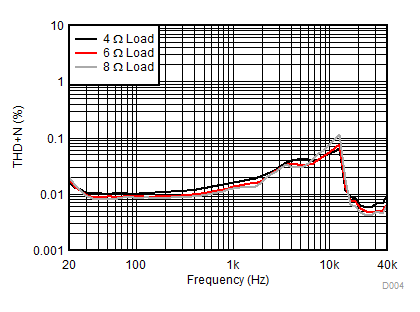AV(SPK_AMP) = 20 dBV PO = 1 W VPVDD = 15 V
Figure 25. THD+N vs Frequency – BTL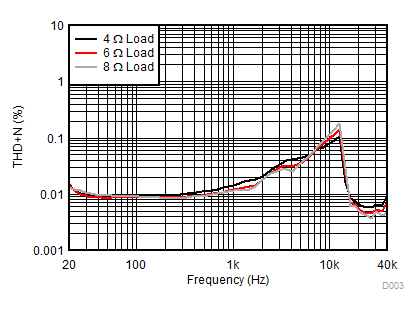AV(SPK_AMP) = 20 dBV PO = 1 W VPVDD = 12 V
Figure 24. THD+N vs Frequency – BTL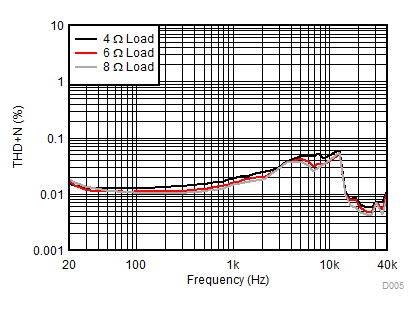AV(SPK_AMP) = 26 dBV PO = 1 W VPVDD = 18 V
Figure 26. THD+N vs Frequency – BTL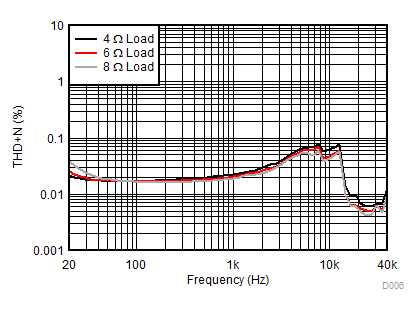AV(SPK_AMP) = 26 dBV PO = 1 W VPVDD = 24 V
Figure 27. THD+N vs Frequency – BTL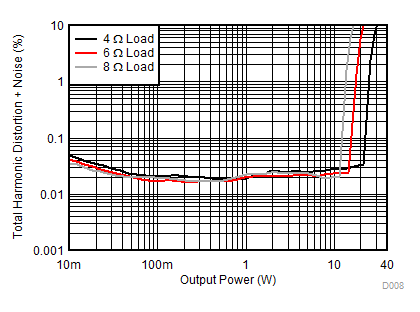AV(SPK_AMP) = 20 dBV VPVDD = 15 V
Figure 29. THD+N vs Power – BTL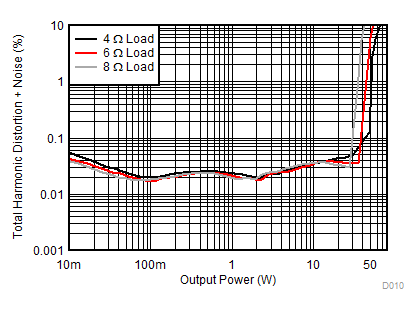AV(SPK_AMP) = 26 dBV VPVDD = 24 V
Figure 31. THD+N vs Power – BTL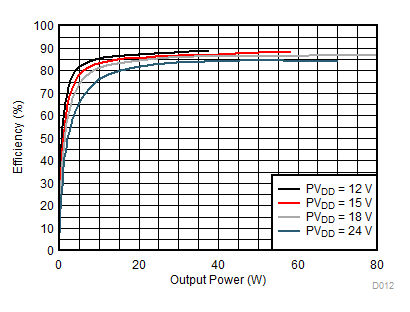RSPK = 4 Ω
Figure 33. Efficiency vs Output Power – BTL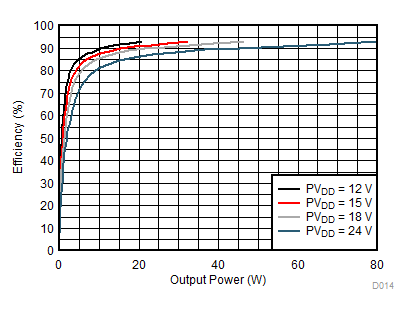RSPK = 8 Ω
Figure 35. Efficiency vs Output Power – BTL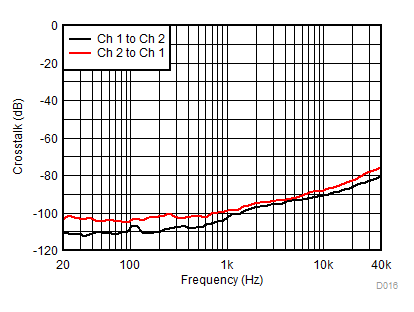AV(SPK_AMP) = 26 dBV VPVDD = 24 V
Figure 37. Crosstalk vs Frequency – BTL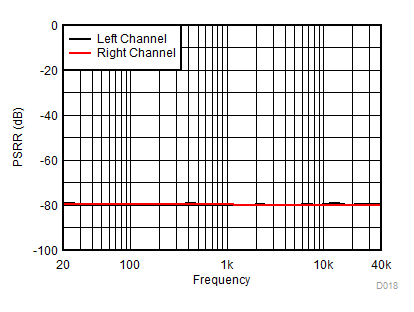AV(SPK_AMP) = 26 dBV VPVDD = 24 V VDVDD = 3.3 V + 250 mVac
Figure 39. DVDD PSRR vs Frequency – BTL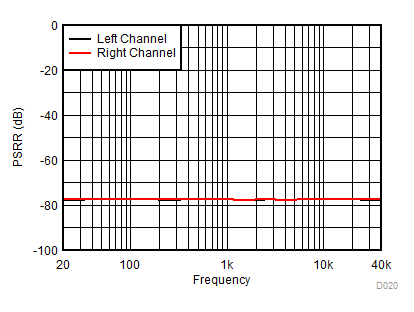AV(SPK_AMP) = 26 dBV VPVDD = 24 V VCPVDD = 3.3 V + 250 mVac
Figure 41. CPVDD PSRR vs Frequency – BTL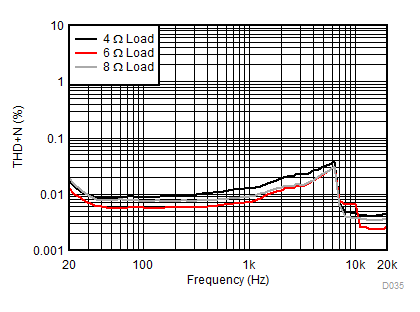AV(SPK_AMP) = 20 dB POUT = 1 W PVDD = 12 V
Figure 43. THD vs Frequency – BTL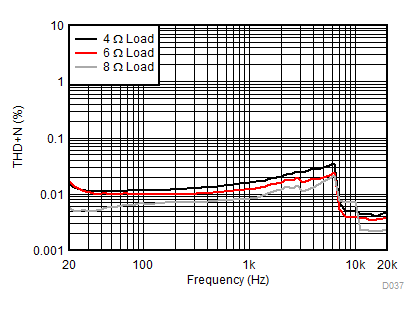AV(SPK_AMP) = 20 dB POUT = 1 W PVDD = 18 V
Figure 45. THD vs Frequency – BTLAV(SPK_AMP) = 20 dBV VPVDD = 12 V
Figure 28. THD+N vs Power – BTL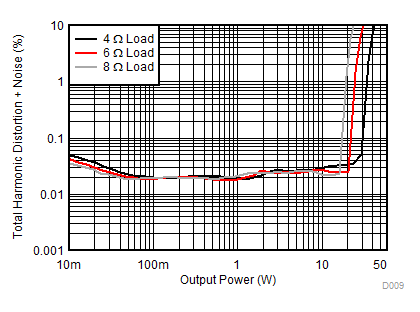AV(SPK_AMP) = 26 dBV VPVDD = 18 V
Figure 30. THD+N vs Power – BTLRSPK = 4 Ω
Figure 32. Idle Channel Noise vs PVDD – BTL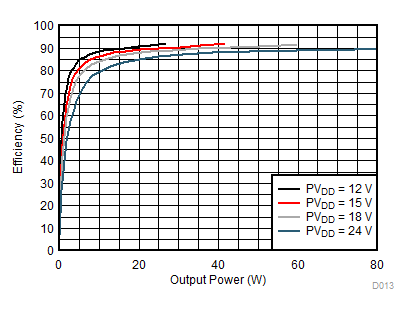RSPK = 6 Ω
Figure 34. Efficiency vs Output Power – BTL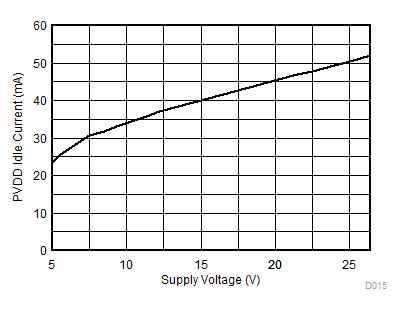fSPK_AMP = 768 kHz RSPK = 8 Ω
Figure 36. Idle Current Draw (Filterless) vs VPVDD – BTL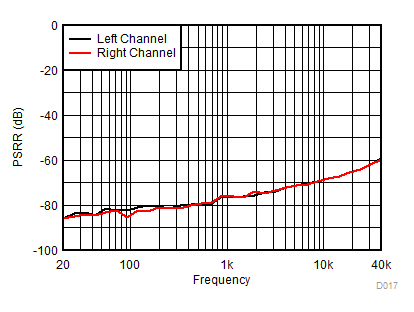AV(SPK_AMP) = 26 dBV VPVDD = 24 V + 250 mVac
Figure 38. PVDD PSRR vs Frequency – BTL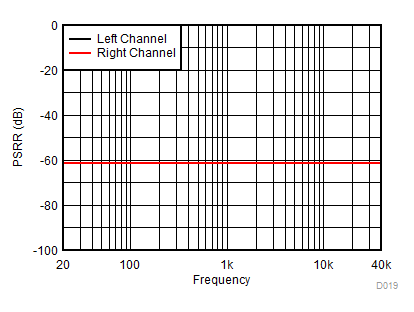AV(SPK_AMP) = 26 dBV VPVDD = 24 V VAVDD = 3.3 V + 250 mVac
Figure 40. AVDD PSRR vs Frequency – BTL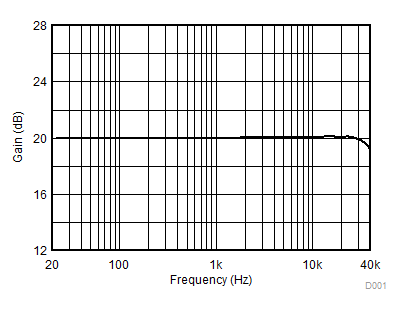AV(SPK_AMP) = 20 dB POUT = 1 W PVDD = 12 V
Figure 42. Gain vs Frequency – BTL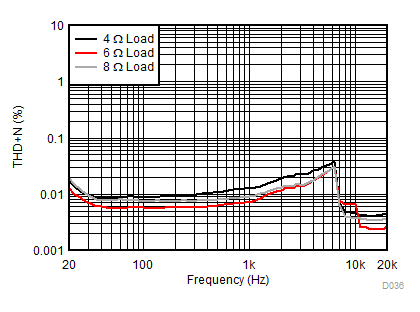AV(SPK_AMP) = 20 dB POUT = 1 W PVDD = 15 V
Figure 44. THD vs Frequency - BTL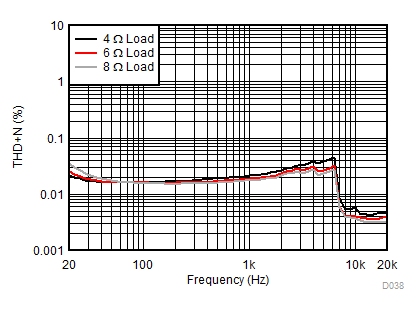AV(SPK_AMP) = 20 dB POUT = 1 W PVDD = 24 V
Figure 46. THD vs Frequency – BTL

7.13.2 Parallel Bridge Tied Load (PBTL) Configuration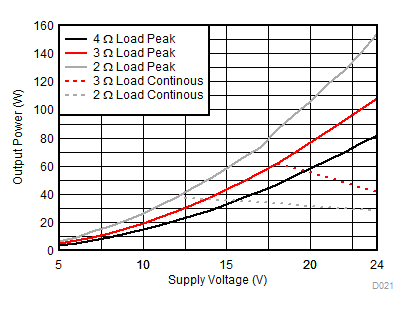AV(SPK_AMP) = 26 dBV
Figure 47. Output Power vs PVDD – PBTL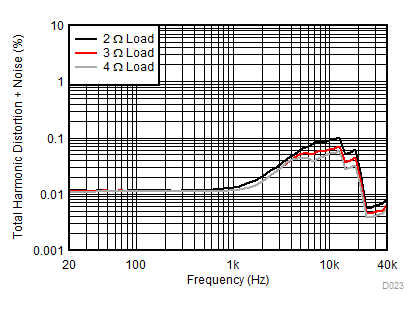AV(SPK_AMP) = 20 dBV PO = 1 W VPVDD = 15 V
Figure 49. THD+N vs Frequency – PBTLAV(SPK_AMP) = 26 dBV PO = 1 W VPVDD = 24 V
Figure 51. THD+N vs Frequency – PBTLAV(SPK_AMP) = 20 dBV VPVDD = 15 V
Figure 53. THD+N vs Power – PBTL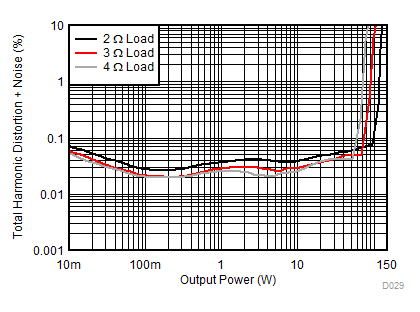AV(SPK_AMP) = 20 dBV VPVDD = 24 V
Figure 55. THD+N vs Power – PBTL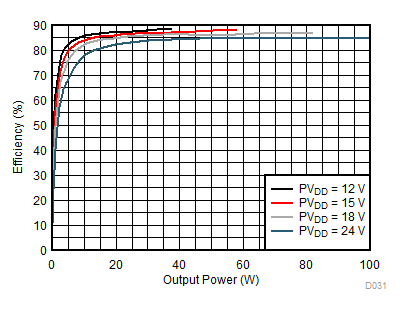AV(SPK_AMP) = 26 dBV RSPK = 2 Ω
Figure 57. Efficiency vs Output Power – PBTL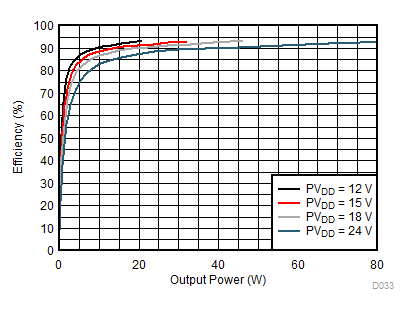AV(SPK_AMP) = 20 dBV RSPK = 4 Ω
Figure 59. Efficiency vs Output Power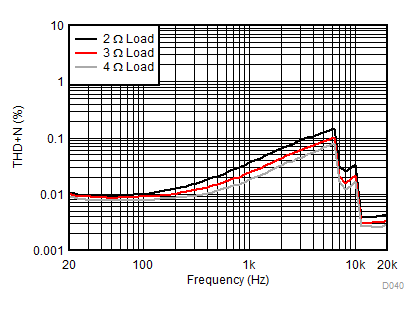AV(SPK_AMP) = 20 dB POUT = 1 W PVDD = 15 V
Figure 61. THD vs Frequency – PBTL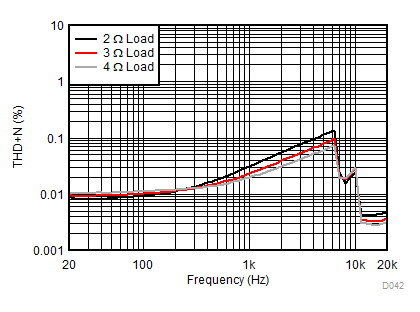AV(SPK_AMP) = 20 dB POUT = 1 W PVDD = 24 V
Figure 63. THD vs Frequency – PBTL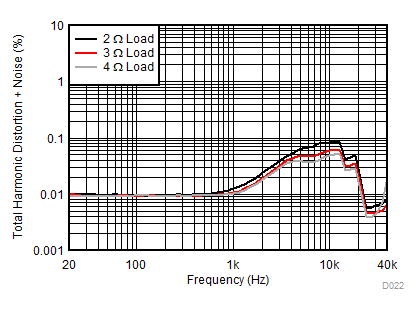AV(SPK_AMP) = 20 dBV PO = 1 W VPVDD = 12 V
Figure 48. THD+N vs Frequency – PBTL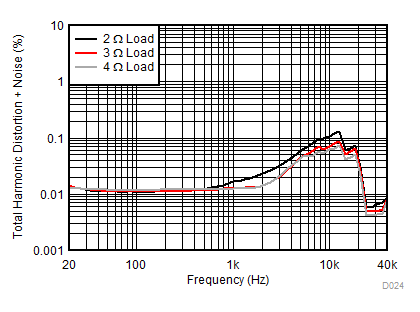AV(SPK_AMP) = 26 dBV PO = 1 W VPVDD = 18 V
Figure 50. THD+N vs Frequency – PBTL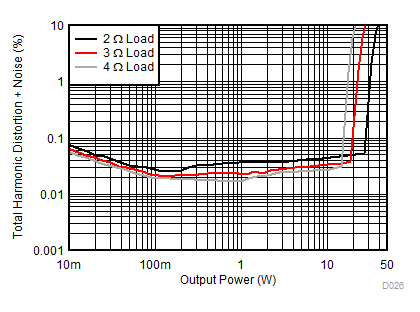AV(SPK_AMP) = 20 dBV VPVDD = 12 V
Figure 52. THD+N vs Power – PBTL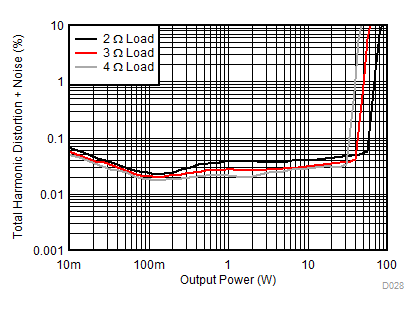AV(SPK_AMP) = 26 dBV VPVDD = 18 V
Figure 54. THD+N vs Power – PBTL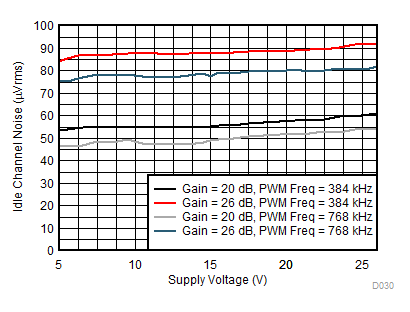RSPK = 4 Ω
Figure 56. Idle Channel Noise vs PVDD – PBTL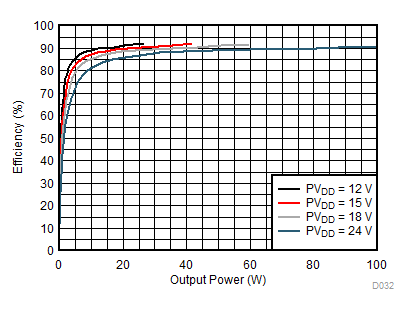AV(SPK_AMP) = 20 dBV RSPK = 3 Ω
Figure 58. Efficiency vs Output Power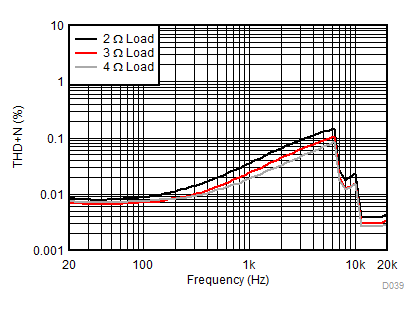AV(SPK_AMP) = 20 dB POUT = 1 W PVDD = 12 V
Figure 60. THD vs Frequency – PBTL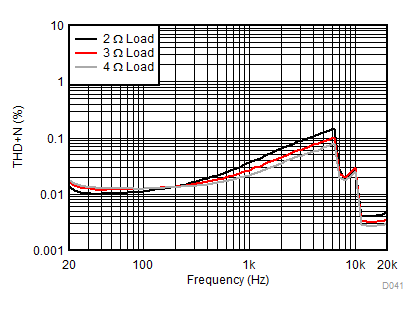AV(SPK_AMP) = 20 dB POUT = 1 W PVDD = 18 V
Figure 62. THD vs Frequency – PBTL